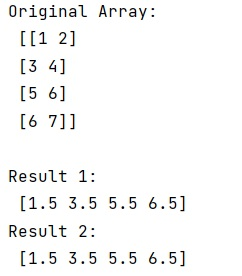# What is the difference between np.mean() and tf.reduce_mean()?

Learn about the difference between np.mean() and tf.reduce_mean() in Python?
Submitted by Pranit Sharma, on January 09, 2023

NumPy is an abbreviated form of Numerical Python. It is used for different types of scientific operations in python. Numpy is a vast library in python which is used for almost every kind of scientific or mathematical operation. It is itself an array which is a collection of various methods and functions for processing the arrays.

## Difference between np.mean() and tf.reduce_mean()

The reduce_mean() in TensorFlow to keep a running average of the results of computations from a batch of inputs.

But, if we are given a list like [1,2,5,4] and we need to compute the mean, we will just pass the whole array to np.mean() and we will get the mean. However, if we need to compute the mean of a stream of numbers, we would have to first assemble the array by reading from the stream and then call np.mean() on the resulting array, we would have to write some more code and hence, as an alternative, we can use the TensorFlow reduce_mean() method.

Let us understand with the help of an example,

## Python code to demonstrate the example of difference between np.mean() and tf.reduce_mean()

```# Import numpy
import numpy as np

# Import tensorflow
import tensorflow as tf

# Creating an array
arr = np.array([[1,2],[3,4], [5,6], [6,7]])

# Display original array
print("Original Array:\n",arr,"\n")

# Calculating mean
res = np.mean(arr,1)

# Display result
print("Result 1:\n",res,"\n")

# Calculating tensorflow reduce_mean
tf_mean = tf.reduce_mean(arr,1)
with tf.Session() as sess:
result = sess.run(tf_mean)

# Display result
print("Result 2:\n",res,"\n")
```

Output: